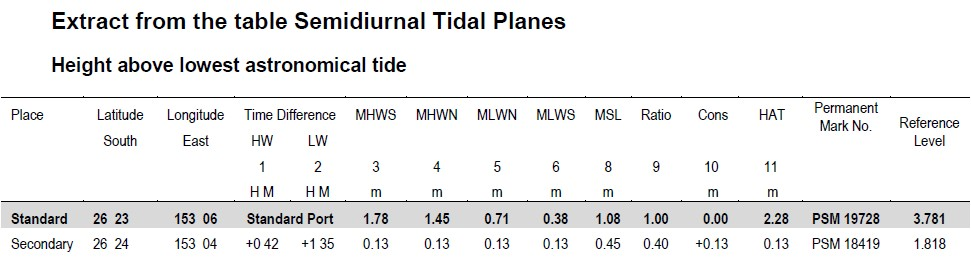# Calculating secondary port tide times

Find the required locality in the table semidiurnal tidal planes or the table diurnal tidal planes and note its standard port.

#### Time of high water

1. Note the time difference in column 1.
2. Add or subtract (as indicated by + or -) this time difference to the predicted time of high water at the standard port.

#### Time of low water

1. Note the time difference in column 2.
2. Add or subtract (as indicated by + or -) this time difference to the predicted time of low water at the standard port.

The result is the approximate time of the tide at the required locality.

#### Height of high water

1. Find the height of the predicted high water at the standard port.
2. Multiply the height by the figure in column 9.
3. Add or subtract (as indicated by the + or -) the figure in column 10.

#### Height of low water

1. Find the height of the predicted low water at the standard port.
2. Multiply the height by the figure in column 9.
3. Add or subtract (as indicated by the + or -) the figure in column 10.#### Example calculation

Find the time and height of high and low tide at a secondary place on the morning of March 16.

Example calculation